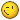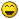# PnCConf spindle max rpm limit issue

27 Mar 2020 01:36 #161652 by cmorley

Dex64 wrote: Here are my config files. Ps. Don't worry about PID settings in XYZ axes I will set it later.Hmm well you didn't mention you were using 2 ranges for spindle control.
the range scaling is all screwed up. seems if pncconf isn't calculating it correctly.
Are you actually using 2 gear ratios?
```#*******************
#  SPINDLE S
#*******************

setp   pid.s.Pgain     [SPINDLE_9]P
setp   pid.s.Igain     [SPINDLE_9]I
setp   pid.s.Dgain     [SPINDLE_9]D
setp   pid.s.bias      [SPINDLE_9]BIAS
setp   pid.s.FF0       [SPINDLE_9]FF0
setp   pid.s.FF1       [SPINDLE_9]FF1
setp   pid.s.FF2       [SPINDLE_9]FF2
net ratio_select.out   pid.s.maxoutput
setp   pid.s.error-previous-target true
setp   pid.s.maxerror .0005

net spindle-index-enable  <=> pid.s.index-enable
net spindle-enable        =>  pid.s.enable
net spindle-vel-cmd-rpm-abs     => pid.s.command
net spindle-vel-fb-rpm-abs      => pid.s.feedback
net spindle-pid-out  pid.s.output    => scale.gear.in
net gear-ratio       ratio_select.out-f => scale.gear.gain
setp ratio_select.in00 0.000167
setp ratio_select.in01 100.000000
net gear-select-a         =>  ratio_select.sel0
net spindle-output        <=  scale.gear.out```

setp ratio_select.in00 0.000167 is not right it should be 1
You haven't connect anything to gear-select-a, o the other ration won't come into affect.

How this works is the requested absolute spindle speed is multiplied by the gear scaling ration then fed to the analog output.
The analog output adds it's own scaling and limts.

What we want is 24000 (requested rpm) x 1 (gear ratio scaling ) x analog scaling = 10volt output

analog scaling works like thus:
[SPINDLE_9]OUTPUT_SCALE = what input RPM gives 10 volt output - so you want 24000 rpm request to be 10 volts - so 24000 is the scale
[SPINDLE_9]OUTPUT_MAX_LIMIT = the maximum input RPM allowed; the component will not increase voltage past this number- so 24000
[SPINDLE_9]OUTPUT_MIN_LIMIT = the minimum input RPM allowed; the component will not decrease voltage under this number - so 0

Of course you may need to tweak the OUTPUT_SCALE a bit to make up for losses/nonlinear output etc but it should be close to this.

Chris
The following user(s) said Thank You: Dex64

27 Mar 2020 02:33 #161655 by cmorley

JohnnyCNC wrote: I have a Huanyang 24K spindle and these are my ini settings. I can't remember where I got the 16800 number from but I can run the spindle up to 400hz 24000 rpm.

[SPINDLE_0]
P = 0
I = 0
D = 0.0
FF0 = 1.00
FF1 = 0.0
FF2 = 0.0
BIAS = 0.0
OUTPUT_MIN_LIMIT = 0
OUTPUT_MAX_LIMIT = 16800
OUTPUT_SCALE = 16800

Was your spindle analog voltage input about 15 volts? 24000/16800 =14.3 volts
on cards like the 7i76 the spindle is controller by a digital pot working on a user selected input voltage between 5 and 15 volts rather then an analog output of +-10 volts.

Chris

You're rightI changed ratio setp ratio_select.in00 0.000167 to 1 and it helped..